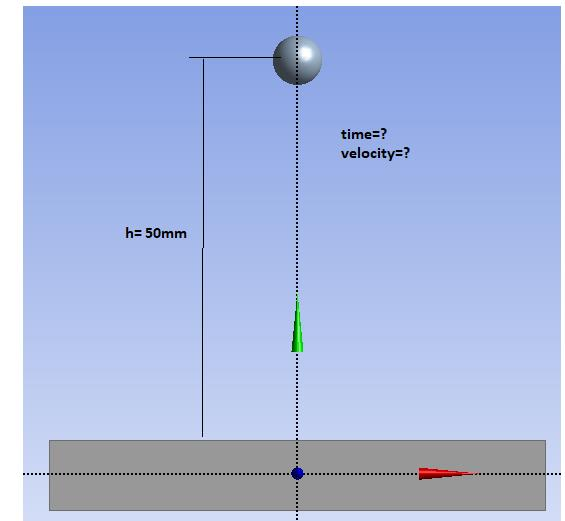## General Mechanical

•Adisa
Subscriber

Hi everybody,

I am trying to do sphere test on the simple . Can anyone has advice for this test. In this example  I do not know time and velocity, the height is known, can I calculate velocity from these equations:

Whether this can be used that calculates free fall sphere from height of 50 mm. From these equations  I can see that the mass has not affect on the magnitude of the velocity. Do I need to use another equation to calculate velocity.

Or can I drop  the sphere nearer the another part for reducing time for solving but that the sphere has the same velocity, which would simulate drop of the sphere from 50mm.

Does anyone know how to do this analysis.Best regards.

•peteroznewman
Subscriber

I have done many simulations of impact due to a sphere being dropped on an object, since it is part of a Safety Standard.

First, measure the drop height, h, from the bottom of the sphere to the surface, not the center.

Second, calculate the impact velocity from an equation with g and h. For h = 0.05 m, v = 0.99 m/s.

Third, use CAD to move the sphere to be tangent to the surface. Check that the initial contact status is closed. Use Adjust to Touch if it isn't.

Fourth, assign the initial velocity calculated in step 2 to the sphere.

In Analysis Settings, select an initial time step to capture the beginning of the compression of the contact area. For example, if the sphere is travelling at 1 m/s and its diameter is 0.005 m, choose 2% of the diameter as the first time step, so a distance of 0.0001 m at 1 m/s takes t = 1e-4 seconds.

ANSYS will automatically increase the time step as the Transient Structural analysis solves.

Best regards.

•Adisa
Subscriber

Peter, thank you for all. You are the best teacher which I know.

Yes, from the link which you posted use the same equations from the first post, but I am now confused.

OK, For Ansys  I understand how to do this analysis, from your steps, but  the velocity from these equation will be the same for difference the sphere, for example: If I am using the sphere with 5 or 15 mm diameter the velocity of the sphere will be the same. The mass do not have affect at the speed of the sphere. Maybe the velocity of the sphere with diameter 15mm will be bigger than with 5mm.

Maybe in Transient Structural need to include Standard Earth Gravity. I do not know, maybe I am wrong.

Thank you.

•peteroznewman
Subscriber

If the 5 and 15 mm start the same distance from the bottom of the sphere to the impact surface, they will have the same velocity. Only if you are talking about a huge height, then mass does make a difference to the terminal velocity where you have to include air resistance.

Yes, you can turn on Standard Earth Gravity. If you simulate for long enough, you can see the sphere rebound, slow down and fall again, but you probably don't care after the sphere has lost contact with the plate after the impact is over.

Best regards.

•Adisa
Subscriber

Yes, You are right, so far i though the sphere with bigger the mass has bigger the  velocity.

I saw a few video, where It is true.

Thank you.

•peteroznewman
Subscriber
•Adisa
Subscriber

Thank Peter,

Does your the first post in this discussion  can be used and for drop test. Or I need to do other set up.

Peter, one more, thank you!

•peteroznewman
Subscriber

The method in my first post in this discussion applied to any drop test simulation.